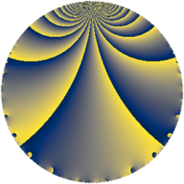# Properties

 Label 7920.2.a.bxLevel $7920$ Weight $2$ Character orbit 7920.a Self dual yes Analytic conductor $63.242$ Analytic rank $0$ Dimension $2$ CM no Inner twists $1$

# Related objects

## Newspace parameters

 Level: $$N$$ $$=$$ $$7920 = 2^{4} \cdot 3^{2} \cdot 5 \cdot 11$$ Weight: $$k$$ $$=$$ $$2$$ Character orbit: $$[\chi]$$ $$=$$ 7920.a (trivial)

## Newform invariants

 Self dual: yes Analytic conductor: $$63.2415184009$$ Analytic rank: $$0$$ Dimension: $$2$$ Coefficient field: $$\Q(\sqrt{2})$$ Defining polynomial: $$x^{2} - 2$$ Coefficient ring: $$\Z[a_1, \ldots, a_{7}]$$ Coefficient ring index: $$1$$ Twist minimal: no (minimal twist has level 3960) Fricke sign: $$-1$$ Sato-Tate group: $\mathrm{SU}(2)$

## $q$-expansion

Coefficients of the $$q$$-expansion are expressed in terms of $$\beta = \sqrt{2}$$. We also show the integral $$q$$-expansion of the trace form.

 $$f(q)$$ $$=$$ $$q - q^{5} + ( 2 + \beta ) q^{7} +O(q^{10})$$ $$q - q^{5} + ( 2 + \beta ) q^{7} + q^{11} -\beta q^{13} -3 \beta q^{17} + ( 4 + 2 \beta ) q^{19} + 2 \beta q^{23} + q^{25} + ( 2 - 4 \beta ) q^{29} + ( 2 - 2 \beta ) q^{31} + ( -2 - \beta ) q^{35} + ( 2 + 4 \beta ) q^{37} + ( 2 + 4 \beta ) q^{41} + ( 2 - 5 \beta ) q^{43} + ( -4 - 6 \beta ) q^{47} + ( -1 + 4 \beta ) q^{49} + ( 2 + 6 \beta ) q^{53} - q^{55} + ( -6 + 2 \beta ) q^{59} + ( 6 - 2 \beta ) q^{61} + \beta q^{65} + 6 \beta q^{67} + ( -2 + 6 \beta ) q^{71} -5 \beta q^{73} + ( 2 + \beta ) q^{77} + ( 8 + 6 \beta ) q^{79} + ( -6 + 3 \beta ) q^{83} + 3 \beta q^{85} + 2 q^{89} + ( -2 - 2 \beta ) q^{91} + ( -4 - 2 \beta ) q^{95} + ( 6 - 2 \beta ) q^{97} +O(q^{100})$$ $$\operatorname{Tr}(f)(q)$$ $$=$$ $$2q - 2q^{5} + 4q^{7} + O(q^{10})$$ $$2q - 2q^{5} + 4q^{7} + 2q^{11} + 8q^{19} + 2q^{25} + 4q^{29} + 4q^{31} - 4q^{35} + 4q^{37} + 4q^{41} + 4q^{43} - 8q^{47} - 2q^{49} + 4q^{53} - 2q^{55} - 12q^{59} + 12q^{61} - 4q^{71} + 4q^{77} + 16q^{79} - 12q^{83} + 4q^{89} - 4q^{91} - 8q^{95} + 12q^{97} + O(q^{100})$$

## Embeddings

For each embedding $$\iota_m$$ of the coefficient field, the values $$\iota_m(a_n)$$ are shown below.

For more information on an embedded modular form you can click on its label.

Label $$\iota_m(\nu)$$ $$a_{2}$$ $$a_{3}$$ $$a_{4}$$ $$a_{5}$$ $$a_{6}$$ $$a_{7}$$ $$a_{8}$$ $$a_{9}$$ $$a_{10}$$
1.1
 −1.41421 1.41421
0 0 0 −1.00000 0 0.585786 0 0 0
1.2 0 0 0 −1.00000 0 3.41421 0 0 0
 $$n$$: e.g. 2-40 or 990-1000 Significant digits: Format: Complex embeddings Normalized embeddings Satake parameters Satake angles

## Atkin-Lehner signs

$$p$$ Sign
$$2$$ $$1$$
$$3$$ $$1$$
$$5$$ $$1$$
$$11$$ $$-1$$

## Inner twists

This newform does not admit any (nontrivial) inner twists.

## Twists

By twisting character orbit
Char Parity Ord Mult Type Twist Min Dim
1.a even 1 1 trivial 7920.2.a.bx 2
3.b odd 2 1 7920.2.a.cf 2
4.b odd 2 1 3960.2.a.u 2
12.b even 2 1 3960.2.a.bb yes 2

By twisted newform orbit
Twist Min Dim Char Parity Ord Mult Type
3960.2.a.u 2 4.b odd 2 1
3960.2.a.bb yes 2 12.b even 2 1
7920.2.a.bx 2 1.a even 1 1 trivial
7920.2.a.cf 2 3.b odd 2 1

## Hecke kernels

This newform subspace can be constructed as the intersection of the kernels of the following linear operators acting on $$S_{2}^{\mathrm{new}}(\Gamma_0(7920))$$:

 $$T_{7}^{2} - 4 T_{7} + 2$$ $$T_{13}^{2} - 2$$ $$T_{17}^{2} - 18$$ $$T_{19}^{2} - 8 T_{19} + 8$$ $$T_{23}^{2} - 8$$ $$T_{29}^{2} - 4 T_{29} - 28$$

## Hecke characteristic polynomials

$p$ $F_p(T)$
$2$ $$T^{2}$$
$3$ $$T^{2}$$
$5$ $$( 1 + T )^{2}$$
$7$ $$2 - 4 T + T^{2}$$
$11$ $$( -1 + T )^{2}$$
$13$ $$-2 + T^{2}$$
$17$ $$-18 + T^{2}$$
$19$ $$8 - 8 T + T^{2}$$
$23$ $$-8 + T^{2}$$
$29$ $$-28 - 4 T + T^{2}$$
$31$ $$-4 - 4 T + T^{2}$$
$37$ $$-28 - 4 T + T^{2}$$
$41$ $$-28 - 4 T + T^{2}$$
$43$ $$-46 - 4 T + T^{2}$$
$47$ $$-56 + 8 T + T^{2}$$
$53$ $$-68 - 4 T + T^{2}$$
$59$ $$28 + 12 T + T^{2}$$
$61$ $$28 - 12 T + T^{2}$$
$67$ $$-72 + T^{2}$$
$71$ $$-68 + 4 T + T^{2}$$
$73$ $$-50 + T^{2}$$
$79$ $$-8 - 16 T + T^{2}$$
$83$ $$18 + 12 T + T^{2}$$
$89$ $$( -2 + T )^{2}$$
$97$ $$28 - 12 T + T^{2}$$